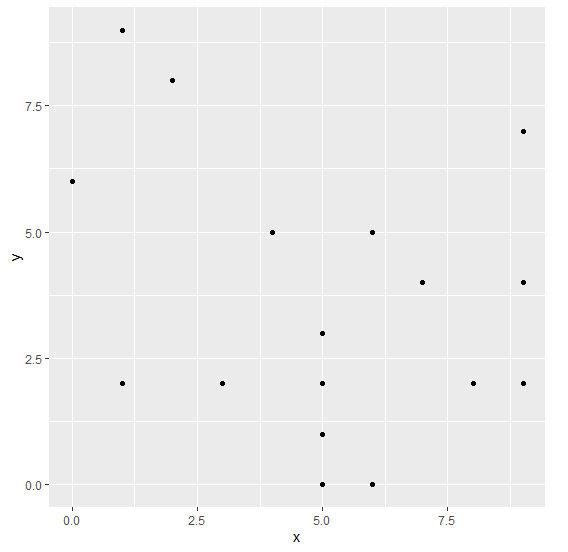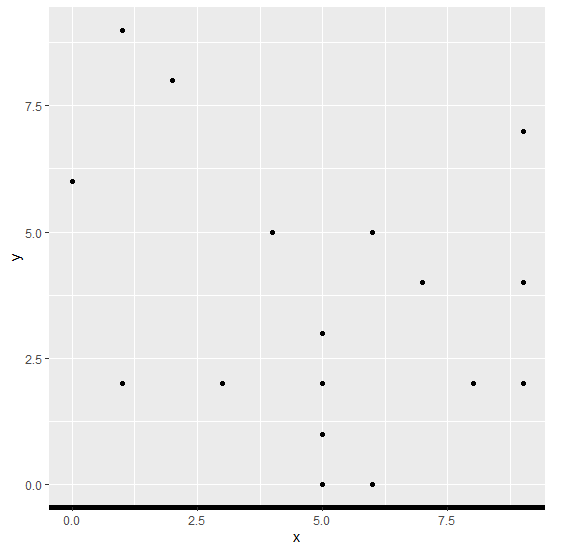# How to increase the width of the X-axis line for a ggplot2 graph?

To increase the width of the X-axis line for a ggplot2 graph in R, we can use theme function where we can set the axis.line.x.bottom argument size to desired size with element_line.

Check out the below Example to understand how it can be done.

## Example

Following snippet creates a sample data frame −

x<-sample(0:9,20,replace=TRUE)
y<-sample(0:9,20,replace=TRUE)
df<-data.frame(x,y)
df

The following dataframe is created

x y
1 4 5
2 5 0
3 5 3
4 7 4
5 1 9
6 0 6
7 6 0
8 9 7
9 6 5
10 5 3
11 2 8
12 3 2
13 5 1
14 1 2
15 8 2
16 6 5
17 5 2
18 1 2
19 9 4
20 9 2

To load ggplot2 package and create scatterplot between x and y on the above created data frame, add the following code to the above snippet −

x<-sample(0:9,20,replace=TRUE)
y<-sample(0:9,20,replace=TRUE)
df<-data.frame(x,y)
library(ggplot2)
ggplot(df,aes(x,y))+geom_point()

## Output

If you execute all the above given snippets as a single program, it generates the following Output −To create scatterplot between x and y with larger width of X-axis line on the above created data frame, add the following code to the above snippet −

x<-sample(0:9,20,replace=TRUE)
y<-sample(0:9,20,replace=TRUE)
df<-data.frame(x,y)
library(ggplot2)
ggplot(df,aes(x,y))+geom_point()+theme(axis.line.x.bottom=element_line(size=2))

## Output

If you execute all the above given snippets as a single program, it generates the following Output −